# Bayesian reasoning

Don’t let the title put you off. This article is about updating your thinking. You may have thought one way about something, such as the probability that you come down with some disease, let’s say, A. Then you get new information in the form of a test for the disease which you take. If the test says you have the disease, does that mean you do? Well, maybe…

First, there is a psychological factor. Maybe you know that, statistically, 20% of people have A at one time or another in their lives. But that’s just a number and you know perfectly well that you don’t have A. So that’s the first result of the test: Because it claims you do have A, you suddenly are catapulted into that part of the statistic, that 20% who “have” A, or so it seems. You have been tested and found wanting.

## Mathematical reasoning

Problem is, such tests are not perfect. They make two kinds of errors: missed positives and false positives. Suppose for this test that it discovers only 80% of the people who have the disease, leaving 20% running around in (happy?) ignorance of their plight. Those 20% are the missed positives. That doesn’t encourage you any. More serious is the number of identification errors the test makes, the false positives. saying that a healthy person has A when she does not. Aha, there is an out, you say. Well, perhaps…

Let’s look at it in a bit more detail. We know the following:

• Only 20% of humanity have A. This is the initial datum you started with..
• Of that 20% who have A, the test finds only 80%, it misses the other 20% completely, the missed positives.
• There are false positives, due to the test’s finding healthy people to be sick. Suppose that is 10%, meaning 10% of the non-sick people.

Let’s run through that one more time, taking a slightly closer look.

• That 20% of humanity have A was all that you knew before you were tested. It is therefore your initial datum, a probability, your opinion before the test. Statisticians call this credence, or degree of confidence.
• The 80% that the test finds. These people all have A and are therefore part of the 20% of the initial datum. This is new information which you must take into account, i.e., learn to live with.
• But 80% of all people do not have A. Nevertheless, the test will find that 10% of them have A anyway, even though that is just plain wrong.

This is easiest to understand if we suppose some real numbers. So, one more round.

• Out of 1,000 people chosen at random, 20% of them have A, i.e., 200 people.
• The test will find only 80% of those, meaning 80% of 200, which is 160 people.
• But of the 800 people who do not have A, the test will claim that 10% of them have A anyway, which is 80 people.

So we have initially 200 people who have A, of whom the test identifies 160. But it also claims that 80 healthy people have A. You want to be in that last group, the healthy ones who test positive. It is easy to calculate the probability of your actually having A. It is just

probability that you have A because the test says you do

= (the number of people the test finds who actually do have A)

divided by

(the total number of people the test says have A, whether they do or not).

If this is not clear, you have the choice of

• going back and reading this all again, or
• giving up, or
• complaining to me that I cannot explain it well enough.1By now, you have figured out that I love lists.

Using P to indicate the probability, the above formula is just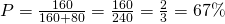Those figures are all large whole numbers because we supposed a sample of 1000 people and multiplied all the probabilities by that number. But since those numbers occur both in the numerator and denominator of our formula, we could divide them out on the top and the bottom and just use the probabilities.

Let’s make sure we understand this.

Initially, you thought the probability of your having A was 20%. Call that probability P(A).

Now, the test has provided you new information which you must use to update your thinking on the matter. But it would be foolish to trust the test completely or forget about that initial estimate.

So let’s take into account both the initial probability P(A) and the test, which we will refer to as B. The new probability you have A is the quotient of the probability that the test has identified you correctly and the probability that it has identified you at all – correctly or incorrectly – call it P(A|B), the funny thingy in parentheses meaning the A probability updated by test B. (Sorry for the notation. If you know better, please let me know.) So the new, updated probability is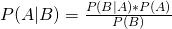(1)

where

• P(A) = the initial probability anyone has A (20%);
• P(B|A) is the probability that someone who has A will be found by the test B (the 80%);
• P(B) is the total probability that anyone, sick or not, will be found by the test (80% of 20% added to 10% of 80%);
• P(A|B) means the probability the test is correct given a positive result of B – and that you have A.

The test is really any new information on the subject. So what this means is that

• P(A) = prior positive confidence (or credence)
• P(-A) = prior negative confidence
• P(B|A) = positive confidence of new information
• P(B|-A) = negative confidence level of new information

(If you got that, skip this parenthetical rehash. If you need more convincing, remember that in our example the 160 is really

• .80 (or 80%), the probability of the test’s identifying a true positive, P(B|A);
• multiplied by .20, the probability of being a true positive before the test. (which you can take to be the “real” proportion of people with A by the initial data), P(A);
• multiplied by 1000, the number of people,

The additional term 80 in the denominator is

• .10 (or 10%), the probability that the test mistakenly identifies a false positive, which we could logically call P(B|-A);
• multiplied by .80 (80%), the probability that anyone does not have A, i.e., 1-P(A).
• multiplied by 1000, the number of people,

End of parenthesis.)

We can make the denominator more explicit by realizing that it is the sum of two terms in this case. Then equation (1) becomes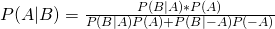(2)

where P(B|-A) is the probability that the test is positive even though one does not have A (10%).

In equation (2), notice what happens if the test is really bad, so that the probability P(B|-A) of false positives is great. The denominator will become very large, so the probability of having A, P(A|B), becomes quite small, as we would expect. Also, if the initial probability of having A, P(A), is very large, then P(B|-A) is small and P(A|B) approaches P(A), again as we expect.

## Back to simple

Whatever be the denominator,

P(A|B) is proportional to the product of P(B|A) and P(A).

The greater the initial probability, the more likely you are to have it; and the greater the accuracy of the test, the same.

Let’s take a silly example. Suppose P(A), the initial opinion, is quite strong, as in the case of a true believer in supernatural phenomena (aka god or gods). Then you reason with him, pointing out that there is no reason whatsoever for accepting such hypotheses. But P(-A) is negligible in his case, so P(A|B) is always about P(A), which is around 100%. You will never convince the guy of anything.

On the other hand, my initial probability for the truth of such myths is P(A) = 0, but let’s be generous and suppose it is 1%, or 0.01. But then P(-A) is huge, around .99, so the denominator blows up and P(A|B) is still around 0. It also helps (or not, according to your point of view) that in this case, the probability of the new information’s being true is also negligible.

A more realistic example, which is often seen, is the case of breast cancer. Here are the probabilities in tabular form.

 Cancer (1%) No cancer (99%) Test positive 80% 9.6% Test negative 20% 90.4%

Most of these probabilities are similar to what we assumed in the first part: 80% of true positives, 20% of false; as well as 9.6% of false positives. But the initial probability is only 1%, not 10%. So we expect a much lower number. What we find is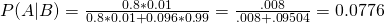i.e., about 7.8%, a rather small probability for having breast cancer. This is understandable, since the test makes rather many mistakes, about 10%, but mainly because the number of women who do not have breast cancer is quite large, about 99%. WARNING: These figures may not be correct, so don’t refuse treatment because of this document: Consult your doctor first.

A last example. In complete ignorance of the facts, let’s suppose that 50% of people over 65 eventually contract AD, Alzheimer’s disease. And let’s suppose that medical ignorance of the subject is such that the only tests are about 50% good, finding half the people who will get it and predicting as many false positives. Then our equation is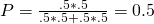or 50%, showing, as we assumed, that we know nothing about it.2I repeat, this is a silly hypothesis and is not true – I hope.

Notes

↑1 By now, you have figured out that I love lists. I repeat, this is a silly hypothesis and is not true – I hope.